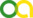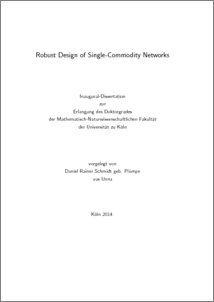Schmidt, Daniel Rainer (2014). Robust Design of Single-Commodity Networks. PhD thesis, Universität zu Köln.Preview
PDF
dissertation-daniel-schmidt.pdf

Abstract

The results in the present work were obtained in a collaboration with Eduardo Álvarez- Miranda, Valentina Cacchiani, Tim Dorneth, Michael Jünger, Frauke Liers, Andrea Lodi and Tiziano Parriani. The subject of this thesis is a robust network design problem, i.e., a problem of the type “dimension a network such that it has sufficient capacity in all likely scenarios.” In our case, we model the network with an undirected graph in which each scenario defines a supply or demand for each node. We say that a flow in the network is feasible for a scenario if it can balance out its supplies and demands. A scenario polytope B defines which scenarios are relevant. The task is now to find integer capacities that minimize the total installation costs while allowing for a feasible flow in each scenario. This problem is called Single-Commodity Robust Network Design Problem (sRND) and was introduced by Buchheim, Liers and Sanità (INOC 2011). The problem contains the Steiner Tree Problem (given an undirected graph and a terminal set, find a minimum cost subtree that connects all terminals) and therefore is N P-hard. The problem is also a natural extension of minimum cost flows. The network design literature treats the case that the scenario polytope B is given as the finite set of its extreme points (finite case) and that it is given as the feasible region of finitely many linear inequalities (polyhedral case). Both descriptions are equivalent, however, an efficient transformation is not possible in general. Buchheim, Liers and Sanità (INOC 2011) propose a Branch-and-Cut algorithm for the finite case. In this case, there exists a canonical problem formulation as a mixed integer linear program (MIP). It contains a set of flow variables for every scenario. Buchheim, Liers and Sanità enhance the formulation with general cutting planes that are called target cuts. The first part of the dissertation considers the problem variant where every scenario has exactly two terminal nodes. If the underlying network is a complete, unweighted graph, then this problem is the Network Synthesis Problem as defined by Chien (IBM Journal of R&D 1960). There exist polynomial time algorithms by Gomory and Hu (SIAM J. of Appl. Math 1961) and by Kabadi, Yan, Du and Nair (SIAM J. on Discr. Math.) for this special case. However, these algorithms are based on the fact that complete graphs are Hamiltonian. The result of this part is a similar algorithm for hypercube graphs that assumes a special distribution of the supplies and demands. These graphs are also Hamiltonian. The second part of the thesis discusses the structure of the polyhedron of feasible sRND solutions. Here, the first result is a new MIP-based capacity formulation for the sRND problem. The size of this formulation is independent of the number of extreme points of B and therefore, it is also suited for the polyhedral case. The formulation uses so-called cut-set inequalities that are known in similar form from other network design problems. By adapting a proof by Mattia (Computational Optimization and Applications 2013), we show that cut-set inequalities induce facets of the sRND polyhedron. To obtain a better linear programming relaxation of the capacity formulation, we interpret certain general mixed integer cuts as 3-partition inequalities and show that these inequalities induce facets as well. The capacity formulation has exponential size and we therefore need a separation algorithm for cut-set inequalities. In the finite case, we reduce the cut-set separation problem to a minimum cut problem that can be solved in polynomial time. In the polyhedral case, however, the separation problem is N P-hard, even if we assume that the scenario polytope is basically a cube. Such a scenario polytope is called Hose polytope. Nonetheless, we can solve the separation problem in practice: We show a MIP based separation procedure for the Hose scenario polytope. Additionally, the thesis presents two separation methods for 3-partition inequalities. These methods are independent of the encoding of the scenario polytope. Additionally, we present several rounding heuristics. The result is a Branch-and-Cut algorithm for the capacity formulation. We analyze the algorithm in the last part of the thesis. There, we show experimentally that the algorithm works in practice, both in the finite and in the polyhedral case. As a reference point, we use a CPLEX implementation of the flow based formulation and the computational results by Buchheim, Liers and Sanità. Our experiments show that the new Branch-and-Cut algorithm is an improvement over the existing approach. Here, the algorithm excels on problem instances with many scenarios. In particular, we can show that the MIP separation of the cut-set inequalities is practical.

Item Type: Thesis (PhD thesis)
Translated abstract:
AbstractLanguage
Die vorliegende Dissertation ist im Rahmen einer Kooperation mit Valentina Cacchiani, Frauke Liers, Andrea Lodi und Michael Jünger entstanden, an der in Teilen auch Eduardo Álvarez-Miranda, Tim Dorneth und Tiziano Parriani beteiligt waren. Das Thema der Arbeit ist ein robustes Netzwerkdesignproblem, d.h. ein Problem der Art „dimensioniere ein Netzwerk so, dass in allen realistischen Szenarien ausreichend Kapazität vorhanden ist.“ In dem konkreten Problem wird das Netzwerk durch einen ungerichteten Graphen modelliert, in dem jedes Szenario einen Angebots- oder Bedarfswert für jeden Knoten definiert. Ein Fluss im Netzwerk heißt zulässig, wenn er alle Angebote und Bedarfe ausgleicht. Welche Szenarien beachtet werden müssen, bestimmt das Szenarienpolytop B. Gesucht sind ganzzahlige Kapazitäten, die zwar die insgesamten Installationskosten min- imieren, aber in jedem Szenario b ∈ B die Existenz eines zulässigen Flusses garantieren. Dieses Single-Commodity Robust Network Design Problem (sRND) geht zurück auf eine Arbeit von Buchheim, Liers und Sanità (INOC 2011) und enthält als Spezialfall das Steiner- baumproblem (gegeben einen ungerichteten Graphen und eine Terminalmenge, finde einen Teilbaum mit minimalen Kosten, der alle Terminale verbindet). Es ist daher N P-schwierig. Außerdem ist es eine natürliche Erweiterung von Minimumkostenflüssen. Die Literatur unterscheidet die Fälle, dass B als endliche Menge seiner Extremalpunkte (endlicher Fall) oder als Lösungsmenge eines linearen Ungleichungssystems gegeben ist (polyedrischer Fall). Diese beiden Fälle sind zwar äquivalent, können jedoch im Allgemeinen nicht effizient ineinander überführt werden. Buchheim, Liers und Sanità (INOC 2011) schlagen einen Branch-and-Cut-Algorithmus für den endlichen Fall vor. In diesem Fall existiert eine kanonische Formulierung des Problems als gemischt-ganzzahliges lineares Programm (MIP). Sie enthält Flussvariablen für jedes Szenario. Um bessere Schranken zu erhalten, fügen Buchheim, Liers und Sanità zusätzlich allgemeine Schnittebenen, sog. Target Cuts, ein. Der erste Teil der Dissertation beschäftigt sich nun mit der Variante des Problems, in der jedes Szenario genau zwei Terminalknoten besitzt. Ist das Netzwerk zusätzlich ein ungewichteter vollständiger Graph, so handelt es sich um das Network Synthesis Problem im Sinne von Chien (IBM Journal of R&D, 1960). Für diesen Fall existieren polynomielle Algorithmen von Gomory und Hu (SIAM J. of Appl. Math. 1961) und Kabadi, Yan, Du und Nair (SIAM J. on Discr. Math. 2009), die jedoch darauf beruhen, dass vollständige Graphen hamiltonsch sind. Wir entwickeln unter der Voraussetzung, dass die Angebote und Bedarfe einer bestimmten Verteilung folgen, einen ähnlicher Algorithmus für Hyperwürfelgraphen. Diese Graphen sind ebenfalls hamiltonsch. Im zweiten Teil der Arbeit geht es um die Struktur des sRND-Lösungspolyeders. Hier ist das erste Ergebnis eine neue MIP-Kapazitätsformulierung des sRND-Problems, deren Größe unabhängig von der Anzahl der Extremalpunkte von B ist. Damit ist die For- mulierung auch für den polyedrischen Fall geeignet. Die Formulierung verwendet sogenannte Cut-Set-Ungleichungen, die in ähnlicher Form auch bei anderen Netzwerkdesignproblemen verwendet werden. Wir wandeln einen Beweis von Mattia (Computational Optimization and Applications 2013) ab und so zeigen so, dass die Cut-Set-Ungleichungen Facetten des Lösungpolyeders sind. Um eine bessere Relaxierung des ganzzahligen linearen Pro- gramms zu erreichen, interpretieren wir bestimmte generische Schnittebenen als sogenannte 3-Partitionsungleichungen und zeigen, dass auch diese Ungleichungen Facetten des Lö- sungspolyeders definieren. Da die Kapazitätsformulierung exponentielle Größe hat, benötigen wir einen Separierungsal- gorithmus für Cut-Set-Ungleichungen. Im endlichen Fall führen wir das Separierungsproblem auf ein Minimum-Schnitt-Problem zurück, das in Polynomialzeit gelöst werden kann. Im polyedrischen Fall ist das Separierungsproblem hingegen N P-schwierig, selbst wenn man davon ausgeht, dass das Szenarienpolytop im Wesentlichen ein Quader – das sogenannte Hose-Polytop – ist. Dennoch kann das Separierungsproblem in der Praxis gelöst werden: Wir zeigen hierzu einen MIP basierten Separierungsansatz für Hose-Szenarienpolytope. Weiterhin stellt die Arbeit zwei Separierungsalgorithmen für 3-Partitionsungleichungen vor, die unabhängig von der Codierung des Szenarienpolytops funktionieren. Zusätzlich führen wir einige Rundungsheuristiken ein. Das Ergebnis ist ein Branch-and-Cut-Algorithmus für die Kapazitätsformulierung, den wir im letzten Teil der Dissertation untersuchen. Dort weisen wir experimentell die praktische Leistungsfähigkeit des Branch-and-Cut-Algorithmus für den endlichen und den polyedrischen Fall nach. Als Referenzpunkt dient dazu eine CPLEX-Implementierung der Flussformulierung und die Rechenergebnisse von Buchheim, Liers und Sanità. Die Experimente zeigen, dass unser neuer Branch-and-Cut-Algorithmus eine Verbesserung des bisherigen Ansatzes darstellt. Dabei eignet er sich vorallem für Probleminstanzen mit vielen Szenarien. Es zeigt sich insbesondere, dass die MIP-Separierung der Schnittungleichungen im polyedrischen Fall auch praktisch anwendbar ist.German
Creators:
CreatorsEmailORCID
Schmidt, Daniel Rainerschmidt@informatik.uni-koeln.deUNSPECIFIED
URN: urn:nbn:de:hbz:38-58727
Subjects: Natural sciences and mathematics
Mathematics
Uncontrolled Keywords:
KeywordsLanguage
Network Design, Robustness, Combinatorial Optimization, Branch-and-Cut, Integer Linear ProgrammingUNSPECIFIED
Faculty: Faculty of Mathematics and Natural Sciences
Divisions: Faculty of Mathematics and Natural Sciences > Institute of Computer Science
Language: English
Date: 2014
Date of oral exam: 1 December 2014
Referee:
Jünger, MichaelProf. Dr.
Liers, FraukeProf. Dr.
Refereed: Yes
URI: http://kups.ub.uni-koeln.de/id/eprint/5872View Item